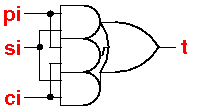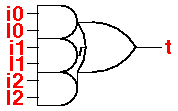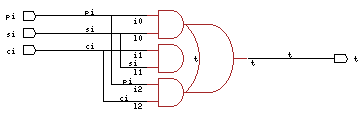A logic macro in BOOG provides a composite primitive function not available in the library, and which will enable BOOG to generate a better netlist. The composite primitive is separated into its library cells when the netlist is read into LOON.The only BOOG macro used here is a non-inverting carry cell.The non-inverting carry cell is called cryb_y and made from the mx3_y which is actually an aon222. A carry cell is an efficient logic function which can produce faster netlists when used. Each pin of the carry cell is connected to two pins of the aon222 cell. We take care that the input pin cap is two pins' worth.Adding this cell improves the critical path of the fastest netlist from 19754 to 18131.

The BOOG macro VBE files are added to the directory MBK_TARGET_LIB pointed to by BOOG. The VST files are put on the search path defined by MBK_CATA_LIB. For this example, MBK_CATA_LIB is

 ```\$ echo \$MBK_CATA_LIB .:\$ALLIANCE_MOS/vbe/sclib100_0\ :\$ALLIANCE_MOS/vbe/sclib_netlist```

The VST files are put into the sclib_netlist directory. Alternative netlists can be tried out by putting them into the working directory (.). A file CATAL must be on the search path and contain the list of primitive cells. I keep this in sclib100_0, but it can be overridden by a file in the working directory if desired.

cryb_y.vbe copied from cry_y.vbe and edited with mx3_y numbers.

 ```ENTITY cryb_y IS GENERIC ( CONSTANT area : NATURAL := 2772; CONSTANT transistors : NATURAL := 18; CONSTANT cin_si : NATURAL := 62; CONSTANT cin_ci : NATURAL := 61; CONSTANT cin_pi : NATURAL := 62; CONSTANT tphh_ci_t : NATURAL := 841; CONSTANT rup_ci_t : NATURAL := 1650; CONSTANT tpll_ci_t : NATURAL := 945; CONSTANT rdown_ci_t : NATURAL := 2310; CONSTANT tphh_pi_t : NATURAL := 858; CONSTANT rup_pi_t : NATURAL := 1650; CONSTANT tpll_pi_t : NATURAL := 913; CONSTANT rdown_pi_t : NATURAL := 2310; CONSTANT tphh_si_t : NATURAL := 721; CONSTANT rup_si_t : NATURAL := 1650; CONSTANT tpll_si_t : NATURAL := 1016; CONSTANT rdown_si_t : NATURAL := 2310 ); PORT ( pi : in BIT; ci : in BIT; si : in BIT; t : out BIT; vdd : in BIT; vss : in BIT ); END cryb_y; ARCHITECTURE VBE OF cryb_y IS BEGIN ASSERT ((vdd and not (vss)) = '1') REPORT "power supply is missing on cryb_y" SEVERITY WARNING t <= (si or ci) and (pi or (si and ci)) after 1000ps; END;```